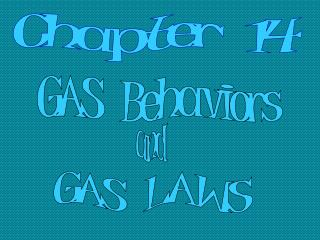DownloadDownload Presentationand

and

Télécharger la présentationand

- - - - - - - - - - - - - - - - - - - - - - - - - - - E N D - - - - - - - - - - - - - - - - - - - - - - - - - - -
Presentation Transcript

1. Chapter 14 GAS Behaviors and GAS LAWS

2. Kinetic Theory of Gases States that gas molecules are in constant motion AND each gas molecule • has no volume as a gas particle • has no attraction between particles • has perfectly elastic collisions (no Energy lost) “Ideal gases” will follow all the gas laws mentioned in Ch. 14 There are no real gases where all these kinetic theory conditions are met perfectly, BUT, at many conditions of temperature and pressure, real gases behave very much like this ideal situation and so assume they are “ideal gases”

3. Four Common Variables used to describe a gas in the equations • P1 and P2 refer to initial and final pressures (in atm or kPa typically) • T1 and T2 refer to initial and final temperatures (in Kelvin for all gas laws) • V1 and V2 refer to initial and final Volumes (in Liters usually) • n refers to the number of moles of gas

4. Avogadro’s Law Avogadro’s law states that equal volumes of any gas at the same temp and pressure have the same # of molecules, and vice versa. Discovered by Amadeo Avogadro and later the 6.02 x 1023 atoms was called “Avogadro’s #” in his honor. Thus a standard conversion between volume, mole and molecules was established at STP (STP is 0ºC and 1atm) 1 trillion molecules of H2(g), He(g) and C3H8(g) all will have equal volumes at the same temp and pressure 22.4Liters of gas at STP = 1 mole = 6.02 x 1023 molecules of gas

5. Boyle's Law: P1V1=P2V2 Initial pressure*initial volume=final pressure*final volume Boyle's Law states that under conditions of constant temperature and quantity of gas, there is an inverse relationship between the volume and pressure for an ideal gas. “More pressure on the system creates less volume”

6. Charles' Law: V1 / T1 = V2 / T2 Charles's Law states that the volume of a given amount of an ideal gas is directly proportional to the Kelvin Temperature provided the amount of gas and the pressure remain fixed. “As temperature increases the volume expands”

7. Gay-Lussac's Law: P1/T1 = P2/T2 This law states that the pressure of a gas is directly proportional to the temperature in degrees Kelvin when the volume is held constant.

8. Combined Gas Law: This equation combines the previous three laws showing the mathematical relationships between the pressure, volume and kelvin temperature of a set quantity of gas as these variables change from the initial to final condition MEMORIZE this one for the test, all the others can be derived from it simply by crossing out one variable on each side (when that variable is constant)

9. Ideal Gas Law: PV=nRT TEMP (k) PRESSURE # OF MOLES VOLUME UNIVERSAL GAS CONSTANT R = .0821 L*atm/ mol* K Or R = 8.31 L*kPa / mol* K Notice that this equation is used only for calculating variables of a gas sample at one point in time, not a before and after scenario.

10. Dalton's Law of Partial Pressures: The total pressure within a closed system can be determined by knowing the partial pressures of each of the constituent gases Total pressure Pt = P1+P2+P3

11. Graham’s Law of Effusion States that the rate of effusion of a gas is inversely proportional to the square root of the gas’s molar mass... large heavy gases move more slowly thru an opening than lighter gases Diffusion = tendency of molecules to move from high to low concentration until equilibrium is reached Effusion = escape of a gas (by diffusion) through a tiny opening

12. What YOU need to Know • You will be able to use the green equation sheet to help you for the next two weeks to do the problem sets I give you (two work packets). • But for the test, no green sheet and no note card • YOU need to memorize • Avogadro’s Law, Graham’s Law, and Dalton’s Law • Combined Gas Law and Ideal Gas Law first • Then be able to derive Boyle’s, Charles’, and Gay-Lussac’s Laws from Combined Law by removing one variable • The test will ask you to recognize whose name is associated with each equation and how to use the equations to solve for unknown variables. The only way to learn this is to practice doing problem sets. Spend time memorizing the equations every night from now until the test.